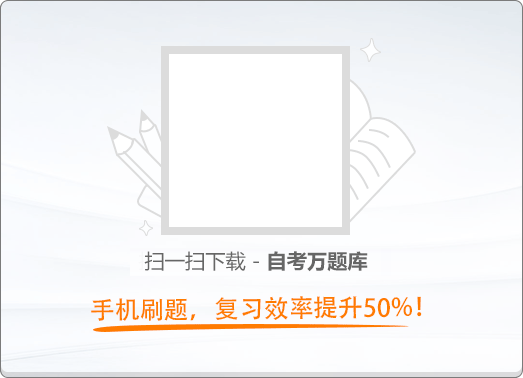150:002018年10月《大学语文》真题

1
(单项选择题)

《寡人之于国也》中，孟子与梁惠王讨论的中心议题是（　）。

• A.

为什么“王好战”

• B.

如何使“民加多”

• C.

不可以“违农时”

• D.

必须要“谨庠序”

• A
• B
• C
• D

2
(单项选择题)

• A.

婵娟

• B.

石秀

• C.

杨雄

• D.

茗烟

• A
• B
• C
• D

3
(单项选择题)

• A.

吃饭有时很像结婚，名义上最主要的东西，其实往往是附属品

• B.

弄饭给我们吃的人，决不是我们真正的主人翁

• C.

整个人世间好比是做菜的厨房

• D.

可口好吃的菜还是值得赞美的

• A
• B
• C
• D

4
(单项选择题)

《冯谖客孟尝君》中，冯援为孟尝君到薛地收债，却尽烧其券，所体现的政治思想是（　）。

• A.

勇于变革

• B.

不甘屈居人下

• C.

无为而治

• D.

注重民心向背

• A
• B
• C
• D

5
(单项选择题)

《张中丞传后叙》写南霁云抽刀断指，“一座大惊，皆感激为云泣下”，这里运用的表现手法是（　）。

• A.

夸张

• B.

铺垫

• C.

衬托

• D.

对比

• A
• B
• C
• D

6
(单项选择题)

• A.

批评长人者政令繁苛

• B.

阐发养民治国思想

• C.

说明种树的正确方法

• D.

赞叹郭橐驼的技艺

• A
• B
• C
• D

7
(单项选择题)

• A.

制国有常，利民为本；从政有经，令行为上

• B.

明德先论于贱，而从政先信于贵

• C.

虽驱世以笑我，胡地、中山，吾必有之

• D.

故寡人变服骑射，欲以备四境之难，报中山之怨

• A
• B
• C
• D

8
(单项选择题)

• A.

天空

• B.

恶鸟

• C.

小青虫

• D.

小粉红花

• A
• B
• C
• D

9
(单项选择题)

《爱尔克的灯光》的主要写作特点是（　）。

• A.

文字简洁省净，情感含蕴多藏，寄深味于平易质朴之中

• B.

熔叙事、写景、记人、抒情于一体，善于铺陈，物象层出

• C.

笔墨客观冷静，写人鲜明生动，剪裁得当，层次井然（面部音项

• D.

熔叙事、抒情、议论于一炉，思绪翻滚，情感浓烈助

• A
• B
• C
• D

10
(单项选择题)

• A.

谈翻译技术

• B.

傅雷夫妇的逝世

• C.

论绘画艺术

• D.

傅雷家书集出版

• A
• B
• C
• D

11
(单项选择题)

《哭小弟》中引用了小弟的诗：“回首悠悠无恨事，丹心一片向将来。”这两句所体现的小弟的美德是（　）。

• A.

不怨天尤人

• B.

不急功近利

• C.

严于律己

• D.

乐于奉献

• A
• B
• C
• D

12
(单项选择题)

《都江堰》：“他大愚，又大智。他大拙，又大巧。他以田间老农的思维，进入了最澄彻的人类学的思考。”这段文字中，“人类学的思考”指的是（　）。

• A.

人们到这儿一次次纳闷：这是谁呢？死于两千年前，却明明还在指挥水流

• B.

他治水的韬略很快被替代成治人的计谋

• C.

没有一个人能活得这样长寿

• D.

这个人种要想不灭绝，就必须要有清泉和米粮

• A
• B
• C
• D

13
(单项选择题)

《蒹葭》形式上的主要特点是（　）。

• A.

全用四言

• B.

重章叠句

• C.

多用对偶

• D.

一韵到底

• A
• B
• C
• D

14
(单项选择题)

《陌上桑》一诗中，“来归相怨怒，但坐观罗敷”的是（　）。

• A.

行者与少年

• B.

耕者与锄者

• C.

使君与吏

• D.

坐中人与夫婚

• A
• B
• C
• D

15
(单项选择题)

《长恨歌》所写唐玄宗与杨贵妃的七夕誓言是（　）。

• A.

后宫佳丽三千人，三千宠爱在一身

• B.

但令心似金钿坚，天上人间会相见

• C.

昭阳殿里恩爱绝，蓬莱宫中日月长

• D.

在天愿作比翼鸟，在地愿为连理枝

• A
• B
• C
• D

16
(单项选择题)

• A.

易安居士

• B.

香山居士

• C.

莲峰居士

• D.

青莲居士

• A
• B
• C
• D

17
(单项选择题)

• A.

有一句话说出就是祸

• B.

别看五千年没有说破

• C.

你猜得透火山的沉默

• D.

突然青天里一个露雳

• A
• B
• C
• D

18
(单项选择题)

• A.

《会唱歌的鸢尾花》

• B.

《双桅船》

• C.

《露珠里的“诗想”》

• D.

《始祖鸟》

• A
• B
• C
• D

19
(单项选择题)

《婴宁》中，王子服初遇婴宁时，婴宁所拈之花为（　）。

• A.

梅花

• B.

杏花

• C.

合欢

• D.

海棠

• A
• B
• C
• D

20
(单项选择题)

《麦琪的礼物》的主旨是（　）。

• A.

谴责不合理的社会等级制度

• B.

反映社会黑暗和人际关系的自私冷漠

• C.

颂扬主人公未经世俗污染的自然天性

• D.

赞美主人公的善良心地和纯真爱情

• A
• B
• C
• D